# 八一干货：如何用高端的方式来说“我爱中国”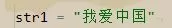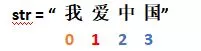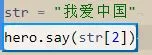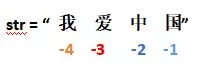``str[-1]``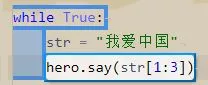``str[1:4]``

``str[-3:]```str="我爱中国"`

`print(str[3:],str[2:],str[1:],str[0:])`

（右滑查看完整代码）

`str="我爱中国"`

`print(str[-1:],str[-2:],str[-3:],str[-4:])`

（右滑查看完整代码）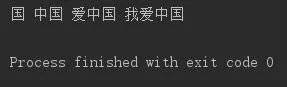（效果相同）

``str = input()``

input()函数的作用十分简单，写上去，用户输入的内容就能被赋入你设置的变量内。

`str=input()`

`forxinrange(len(str)):`

`    print(str[-(x+1):])`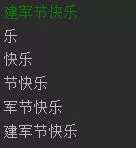Tips

`str=input()`

`[print(str[-(x+1):])forxinrange(len(str))]`